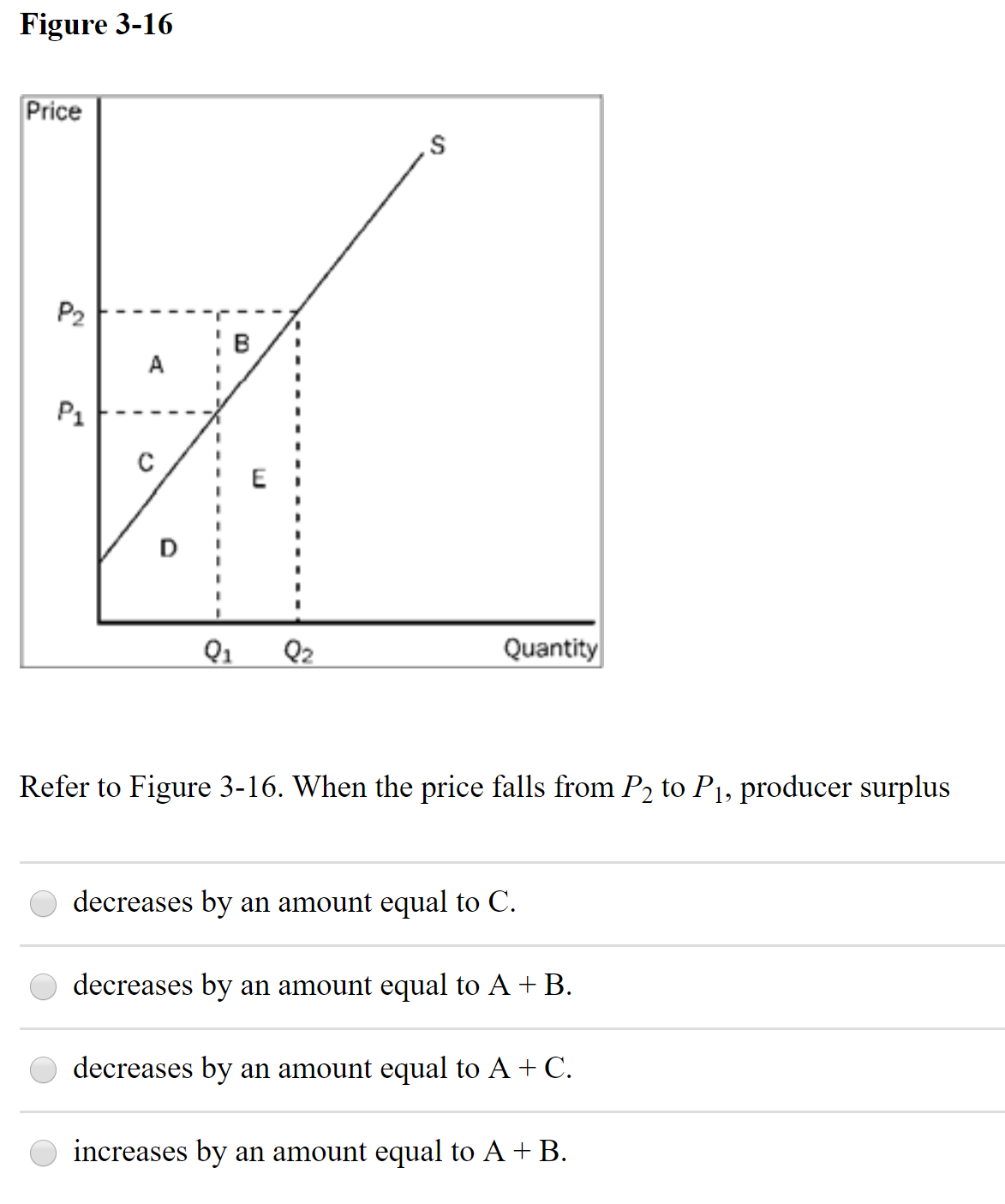# Figure 3-16PriceP2AP1Q1Q2QuantityRefer to Figure 3-16. When the price falls from P2 to P1, producer surplusdecreases by an amount equal to C.decreases by an amount equal to A + B.decreases by an amount equal to A + C.increases by an amount equal to A + B.

Question
6 viewshelp_outlineImage TranscriptioncloseFigure 3-16 Price P2 A P1 Q1 Q2 Quantity Refer to Figure 3-16. When the price falls from P2 to P1, producer surplus decreases by an amount equal to C. decreases by an amount equal to A + B. decreases by an amount equal to A + C. increases by an amount equal to A + B. fullscreen
check_circle

Step 1

Graphically producer surplus is the area lies below the price line and above the supply curve.

Producer surplus w...

### Want to see the full answer?

See Solution

#### Want to see this answer and more?

Solutions are written by subject experts who are available 24/7. Questions are typically answered within 1 hour.*

See Solution
*Response times may vary by subject and question.
Tagged in